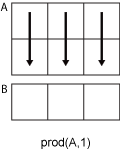# prod

## 语法

``B = prod(A)``
``B = prod(A,'all')``
``B = prod(A,dim)``
``B = prod(A,vecdim)``
``B = prod(___,outtype)``
``B = prod(___,nanflag)``

## 说明

````B = prod(A)` 返回 `A` 的数组元素的乘积。 如果 `A` 是向量，则 `prod(A)` 返回元素的乘积。如果 `A` 为非空矩阵，则 `prod(A)` 将 `A` 的各列视为向量，并返回一个包含每列乘积的行向量。 如果 `A` 为 0×0 空矩阵，`prod(A)` 返回 `1`。如果 `A` 为多维数组，则 `prod(A)` 沿第一个非单一维度运算并返回乘积数组。此维度的大小将减少至 `1`，而所有其他维度的大小保持不变。 如果输入 `A` 为 `single` 类型，则 `prod` 会计算并将 `B` 以 `single` 类型返回。如果为任何其他数值和逻辑数据类型，`prod` 会计算并将 `B` 以 `double` 类型返回。```

``B = prod(A,'all')` 计算 `A` 的所有元素的乘积。此语法适用于 MATLAB® R2018b 及更高版本。`

``B = prod(A,dim)` 返回沿维度 `dim` 的乘积。例如，如果 `A` 为矩阵，则 `prod(A,2)` 为一个包含每一行乘积的列向量。`

``B = prod(A,vecdim)` 根据向量 `vecdim` 中指定的维度计算乘积。例如，如果 `A` 是矩阵，则 `prod(A,[1 2])` 是 `A` 中所有元素的乘积，因为矩阵的每个元素都包含在由维度 1 和 2 定义的数组切片中。`

``B = prod(___,outtype)` 使用前面语法中的任何输入参数按 `outtype` 指定的类返回数组。`outtype` 可以是 `'double'`、`'native'` 或 `'default'`。`

``B = prod(___,nanflag)` 指定在上述任意语法的计算中是包括还是忽略 `NaN` 值。`prod(A,'includenan')` 会在计算中包括 `NaN` 值，而 `prod(A,'omitnan')` 则忽略这些值。`

## 示例

`A=[1:3:7;2:3:8;3:3:9]`
```A = 3×3 1 4 7 2 5 8 3 6 9 ```

`B = prod(A)`
```B = 1×3 6 120 504 ```

`A = [true false; true true]`
```A = 2x2 logical array 1 0 1 1 ```

`B = prod(A)`
```B = 1×2 1 0 ```

`class(B)`
```ans = 'double' ```

`A=[1:3:7;2:3:8;3:3:9]`
```A = 3×3 1 4 7 2 5 8 3 6 9 ```

```dim = 2; B = prod(A,dim)```
```B = 3×1 28 80 162 ```

```A(:,:,1) = [2 4; -2 1]; A(:,:,2) = [1 2; -5 3]; A(:,:,3) = [4 4; 1 -3]; B1 = prod(A,[1 2])```
```B1 = B1(:,:,1) = -16 B1(:,:,2) = -30 B1(:,:,3) = -48 ```

`B2 = prod(A,[1 2 3])`
```B2 = -23040 ```
`Ball = prod(A,'all')`
```Ball = -23040 ```

`A = single([1200 1500 1800; 1300 1600 1900; 1400 1700 2000])`
```A = 3x3 single matrix 1200 1500 1800 1300 1600 1900 1400 1700 2000 ```

`B = prod(A,2,'double')`
```B = 3×1 109 × 3.2400 3.9520 4.7600 ```

`class(B)`
```ans = 'double' ```

`A = uint8([1:3:7;2:3:8;3:3:9])`
```A = 3x3 uint8 matrix 1 4 7 2 5 8 3 6 9 ```

`B = prod(A,'native')`
```B = 1x3 uint8 row vector 6 120 255 ```

`class(B)`
```ans = 'uint8' ```

```A = [1 3 2 4 NaN 3 NaN 2]; P = prod(A,'omitnan')```
```P = 144 ```

## 输入参数

• 如果 `dim = 1`，则 `prod(A,1)` 返回包含每一列中元素的乘积的行向量。• 如果 `dim = 2`，则 `prod(A,2)` 返回包含每一行中元素的乘积的列向量。`dim` 大于 `ndims(A)` 时，`prod` 返回 `A``outtype`输出数据类型
`'default'``double`，除非输入数据类型为 `single`。在这种情况下，输出数据类型为 `single`
`'double'``double`
`'native'`与输入数组 `A` 相同的数据类型

`NaN` 条件，指定为下列值之一：

• `'includenan'` - 计算乘积时包括输入中的 `NaN` 值，其对应输出为 `NaN` 值。

• `'omitnan'` - 忽略输入中的 `NaN` 值。如果所有元素均为 `NaN`，则 `prod` 返回 1。

## 输出参数

`B` 的类如下所示：

• 如果 `outtype` 参数指定为 `'default'` 或未使用它

• 并且输入不是 `single`，则输出为 `double`

• 并且输入为 `single`，则输出为 `single`

• 如果 `outtype` 参数指定 `'double'`，则输出为 `double`，这与输入的数据类型无关。

• 如果 `outtype` 参数指定 `'native'`，则输出与输入具有相同的数据类型。

## 详细信息

### 第一个非单一维度

• 如果 `X` 为 1×n 的行向量，则第二个维度是 `X` 的第一个非单一维度。

• 如果 `X` 为 1×0×n 的空数组，则第二个维度是 `X` 的第一个非单一维度。

• 如果 `X` 为 1×1×3 的数组，则第三个维度是 `X` 的第一个非单一维度。